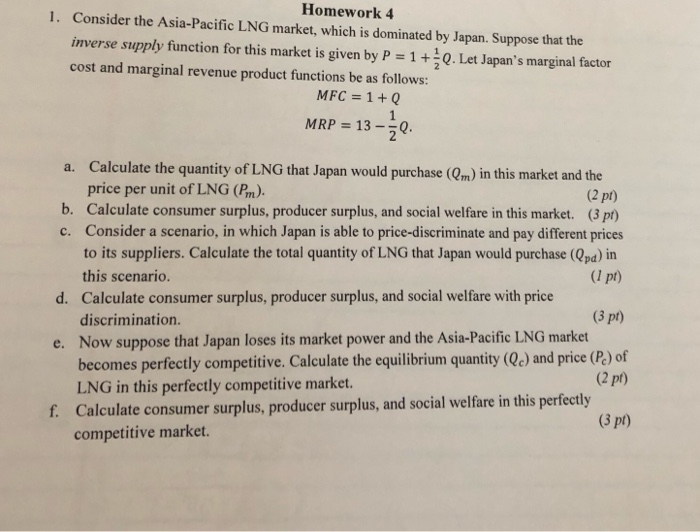# Homework 4 1. Consider the Asia-Pacific LNG market, which is dominated by Japan. Suppose that the...Homework 4 1. Consider the Asia-Pacific LNG market, which is dominated by Japan. Suppose that the inverse supply function for this market is given by P 1.Let Japan's marginal factor cost and marginal revenue product functions be as follows: MFC 1 +Q MRP 13- Calculate the quantity of LNG that Japan would purchase (Qm) in this market and the price per unit of LNG (Pm) a. (2 pt) b. Calculate consumer surplus, producer surplus, and social welfare in this market. (3pt) c. Consider a scenario, in which Japan is able to price-discriminate and pay different prices to its suppliers. Calculate the total quantity of LNG that Japan would purchase (Qpa) in this scenario. Calculate consumer surplus, producer surplus, and social welfare with price discrimination. Now suppose that Japan loses its market power and the Asia-Pacific LNG marker becomes perfectly competitive. Calculate the equilibrium quantity (Qe) and price (P) of LNG in this perfectly competitive market. Calculate consumer surplus, producer surplus, and social welfare in this perfectly (I pt) d. 3 pt) e. (2 pt) f. 3 pt) competitive market.

a) Japan would purchase in this market when MFC = MRC( increase in cost because extra unit is equal to incremental revenue by selling that unit

Therefore,

1+Q = 13-1/2Q

= Q = 8

Putting this value of Q in supply function we get the price

P = 1+1/2*(8) = 5

Japan would purchase 8 units at a price of \$5/unit

b)

##### Add Answer of: Homework 4 1. Consider the Asia-Pacific LNG market, which is dominated by Japan. Suppose that the...
More Homework Help Questions Additional questions in this topic.

• #### out of the four economic market models : competitive market, monopoly market, monopolistic competition and oligopolywhich is the best market model and which is the worst

Need Online Homework Help?